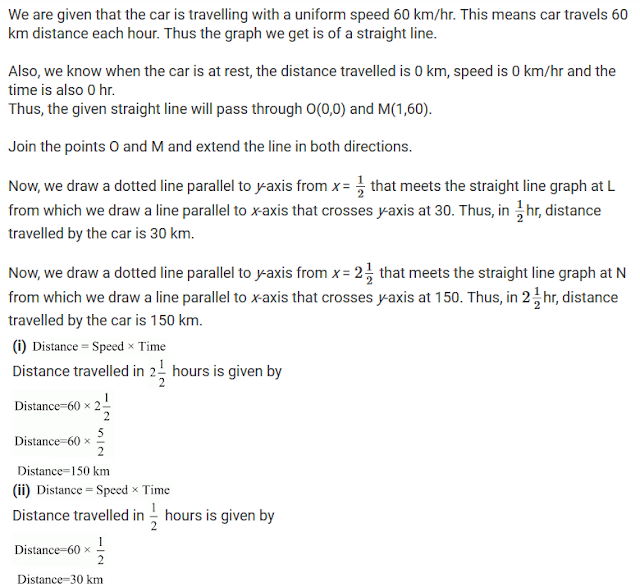#### Chapter 13 Linear Equations in Two Variables R.D. Sharma Solutions for Class 9th Exercise 13.3

Exercise 13.3

1. Draw the graph of each of the following linear equations in two variables:
(i) x+y = 4
(ii) x-y = 0
(iii) -x+y = 6
(iv) y = 2x
(v) 3x+5y = 15
(vi) x/2 - y/3 = 2
(vii) (x-2)/3 = y-3
(viii) 2y = -x+1

Solution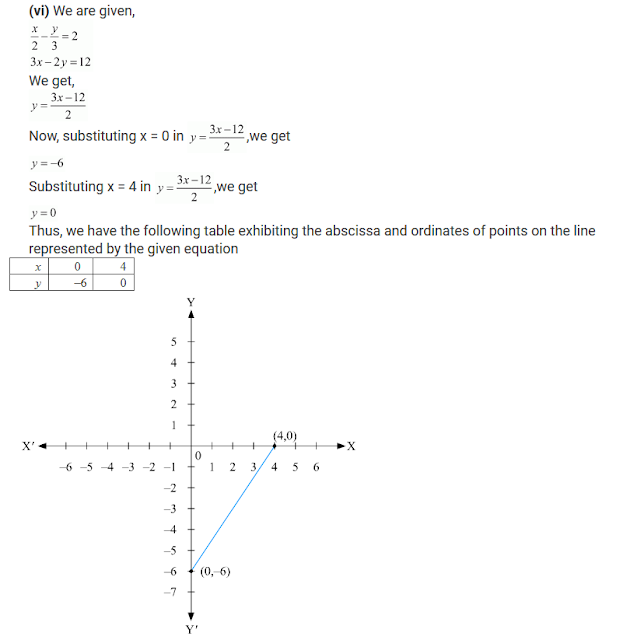2 . Give the equations of two lines passing through (3, 12). How many more such lines are there, and why?

Solution

We observe that x = 3 and y = 12 is the solution of the following equations
4x-y = 0 and 3x-y+3 = 0
So, we get the equations of two lines passing through (3, 12) are, 4x – y = 0 and 3x – y + 3 = 0.
We know that passing through the given point infinitely many lines can be drawn. So, there are infinitely many lines passing through (3,12)

3. A three-wheeler scooter charges Rs 15 for first kilometer and Rs 8 each for every subsequent kilometer. For a distance of x km, an amount of Rs y is paid. Write the linear equation representing the above information.

Solution

Total fare of Rs y for covering the distance of x km is given by
y = 15 + 8(x − 1)
y = 15 + 8x − 8
y = 8x + 7
Where, Rs y is the total fare (x – 1) is taken as the cost of first kilometer is already given Rs 15 and 1 has to subtracted from the total distance travelled to deduct the cost of first kilometer.

4. A lending library has a fixed charge for the first three days and an additional charge for each day thereafter. Aarushi paid Rs 27 for a book kept for seven days. If fixed charges are Rs x and per day charges are Rs y. Write the linear equation representing the above information.

Solution
Total charges of Rs 27 of which Rs x for first three days and Rs y per day for 4 more days is given by
x+y(7-3) = 27
⇒ x+4y = 27
⇒ x+y(7-3)=27
⇒ x+4y=27
Here, (7, -3) is taken as the charges for the first three days are already given at Rs x and we have to find the charges for the remaining four days as the book is kept for the total of 7 days.

5. A number is 27 more than the number obtained by reversing its digits. If its unit's and ten's digit are x and y respectively, write the linear equation representing the above statement.

Solution

The number given to us is in the form of yx,
where y represents the ten's place of the number and x represents the unit's place of the number.
Now,
Given number is 10y + x
Number obtained by reversing the digits of the number is 10x + y
It is given to us that the original number is 27 more than the number obtained by reversing its digits
So,
10y + x = 10x + y +27
⇒ 10y- y+x-10x = 27
⇒ 9y-9x = 27
⇒ 9(y- x) = 27
⇒ y-x = 27/9
⇒ y-x=3

6. The sum of a two digit number and the number obtained by reversing the order of its digits is 121. If units and ten's digit of the number are x and y respectively, then write the linear equation representing the above statement.

Solution7. Plot the points (3,5) and (−1, 3) on a graph paper and verify that the straight line passing through these points also passes through the point (1, 4).

Solutions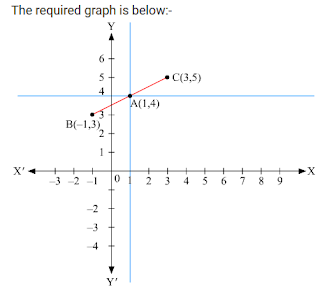By plotting the given points (3, 5) and (–1, 3) on a graph paper, we get the line BC.
We have already plotted the point A (1, 4) on the given plane by the intersecting lines.
Therefore, it is proved that the straight line passing through (3, 5) and (–1, 3) also passes through A (1, 4).

8. From the choices given below, choose the equation whose graph is given in Fig. 13.13.
(i) y = x
(ii) x + y = 0
(iii) y = 2x
(iv) 2 + 3y = 7xSolution

We are given co-ordinates (1, –1) and (–1, 1) as the solution of one of the following equations.
We will substitute the value of both co-ordinates in each of the equation and find the equation which satisfies the given co-ordinates.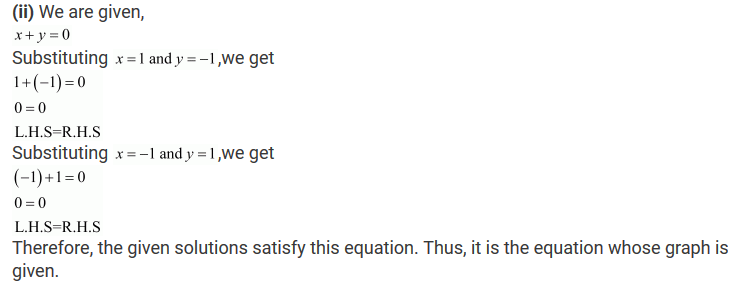9. From the choices given below, choose the equation whose graph is given in Fig. 13.14.

(i) y= x + 2
(ii) y = x −2
(iii) y = x + 2
(iv) x + 2y = 6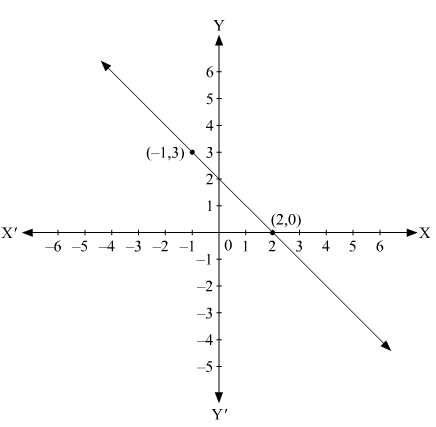Solution

We are given co-ordinates (–1, 3) and (2, 0) as the solution of one of the following equations.
We will substitute the value of both co-ordinates in each of the equation and find the equation which satisfies the given co-ordinates.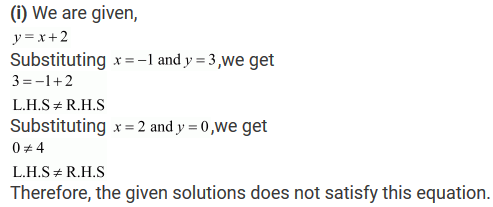10. If the point (2, −2) lies on the graph of the linear equation 5x + ky = 4, find the value of k.

Solution11. Draw the graph of the equation 2x + 3y = 12. From the graph, find the coordinates of the point:
(i) whose y-coordinates is 3.
(ii) whose x-coordinate is -3.

Solution12. Draw the graph of each of the equations given below. Also, find the coordinates of the points where the graph cuts the coordinate axes:
(i) 6x − 3y = 12
(ii) -x + 4y = 8
(iii) 2x + y = 6
(iv) 3x + 2y + 6 = 0

Solution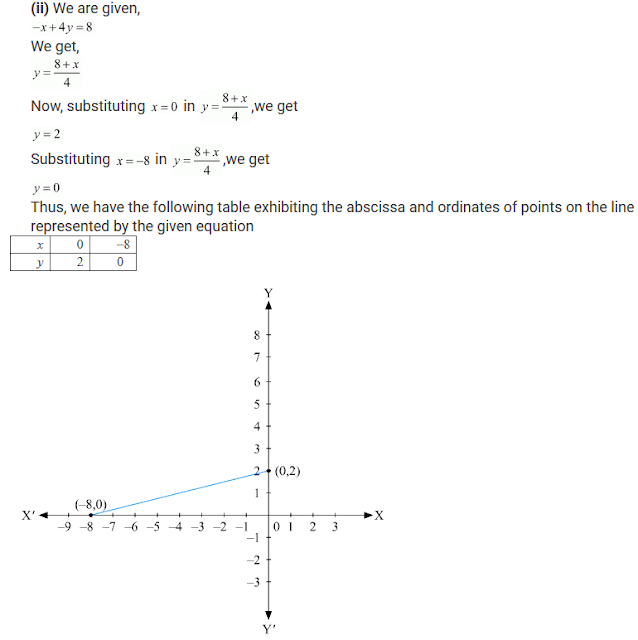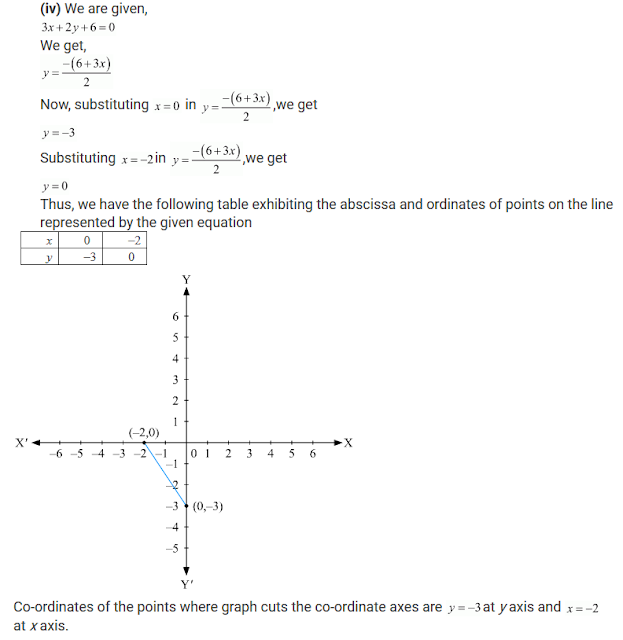13. Draw the graph of the equation 2x + y = 6. Shade the region bounded by the graph and the coordinate axes. Also, find the area of the shaded region.

Solution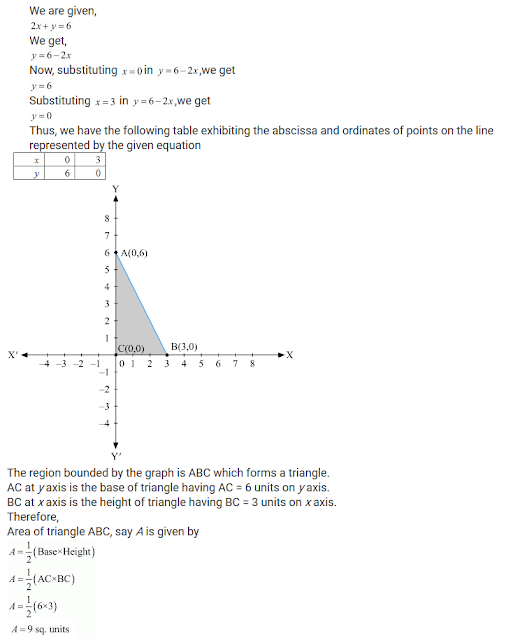14. Draw the graph of the equation x/3 + y/4 = 1. Also, find the area of the triangle formed by the line and the coordinates axes.

Solution15. Draw the graph of y = | x |.

Solution16. Draw the graph of y = | x | + 2.

Solution17. Draw the graphs of the following linear equations on the same graph paper:
2x + 3y = 12, x − y = 1
Find the coordinates of the vertices of the triangle formed by the two straight lines and the area bounded by these lines and x-axis.

Solution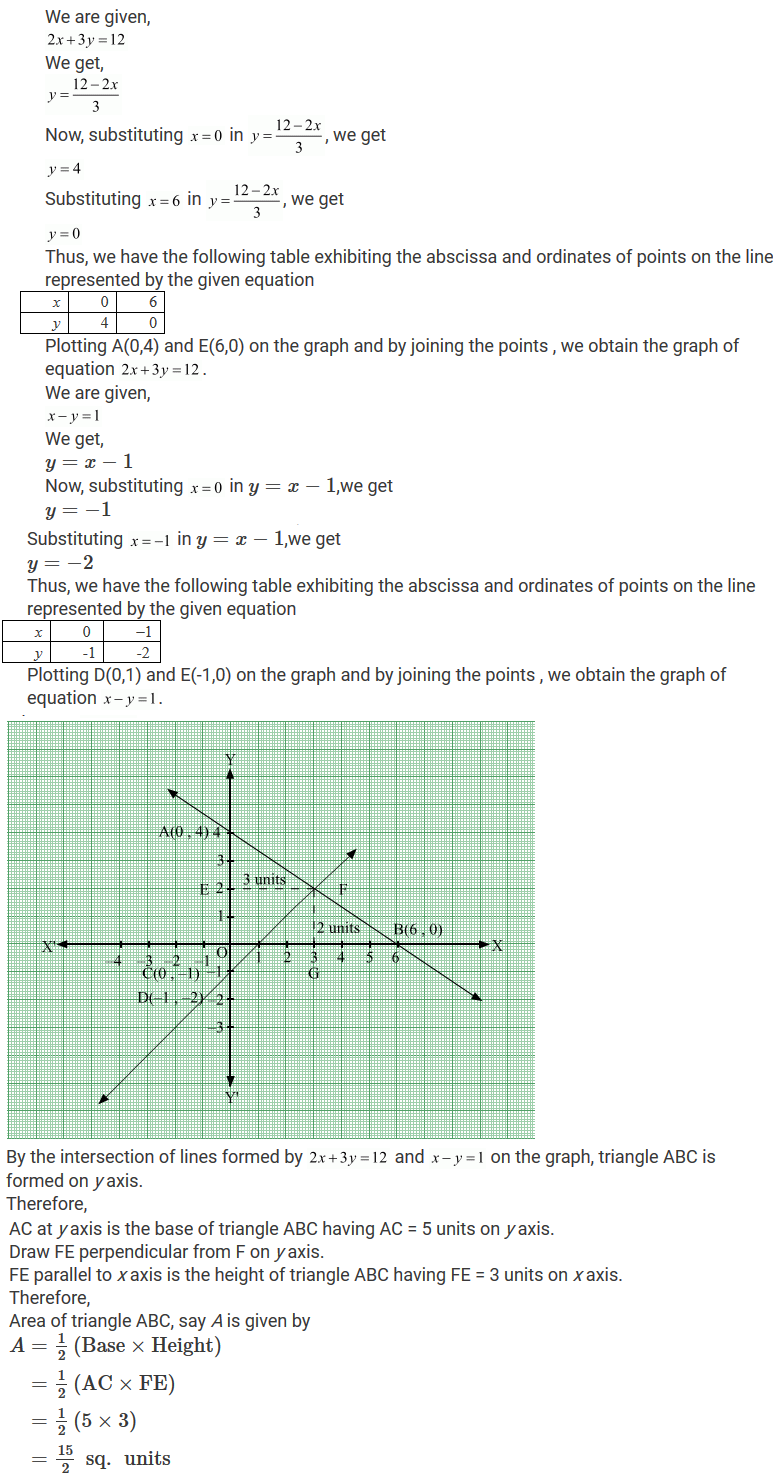18. Draw the graphs of the linear equations 4x − 3y + 4 = 0 and 4x + 3y −20 = 0. Find the area bounded by these lines and x-axis.

Solution19. The path of a train A is given by the equation 3x + 4y − 12 = 0 and the path of another train B is given by the equation 6x + 8y − 48 = 0. Represent this situation graphically.

Solution20. Ravish tells his daughter Aarushi, ''Seven years ago, I was seven times as old as you were then. Also, three years from now, I shall be three times as old as you will be''. If present ages of Aarushi and Ravish are x and y years respectively, represent this situation algebraically as well as graphically.

Solution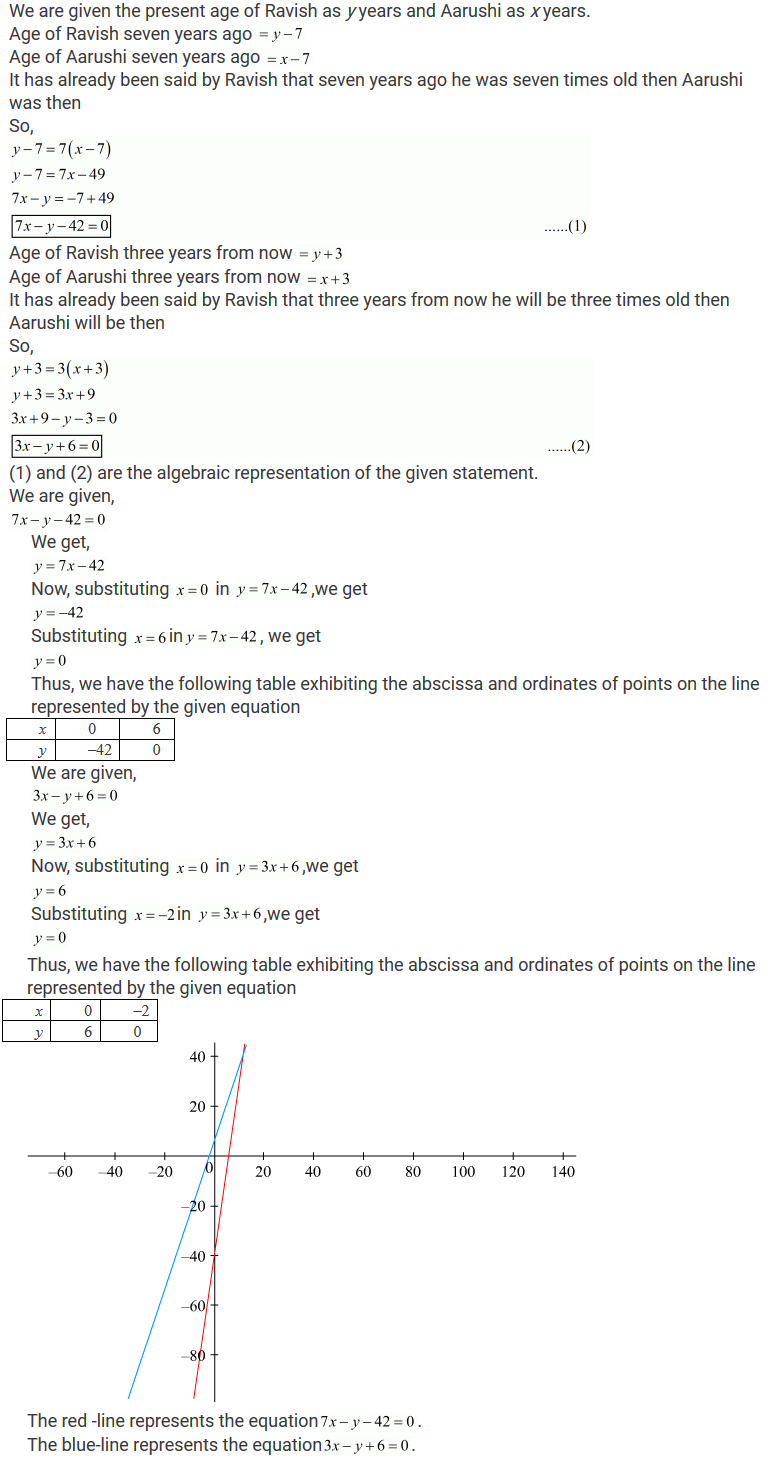21. Aarushi was driving a car with uniform speed of 60 km/h. draw distance-time graph. From the graph, find the distance travelled by Aarushi in
(i) 2½ Hours
(ii) 1/2 Hour

Solution

Aarushi is driving the car with the uniform speed of 60 km/h.
We represent time on X-axis and distance on Y-axis
Now, graphically